Updating search results...

# 44 Results

View
Selected filters:
• exponents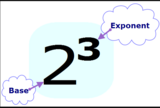Conditional Remix & Share Permitted
CC BY-NC
Rating
0.0 stars

Students&nbsp;understand the meaning of exponents.&nbsp;&nbsp;

Subject:
Mathematics
Material Type:
Lesson Plan
Author:
Angela Vanderbloom
07/04/2018Conditional Remix & Share Permitted
CC BY-NC-SA
Rating
0.0 stars

In earlier modules, students analyze the process of solving equations and developing fluency in writing, interpreting, and translating between various forms of linear equations (Module 1) and linear and exponential functions (Module 3). These experiences combined with modeling with data (Module 2), set the stage for Module 4. Here students continue to interpret expressions, create equations, rewrite equations and functions in different but equivalent forms, and graph and interpret functions, but this time using polynomial functions, and more specifically quadratic functions, as well as square root and cube root functions.

Find the rest of the EngageNY Mathematics resources at https://archive.org/details/engageny-mathematics.

Subject:
Algebra
Mathematics
Material Type:
Module
Provider:
New York State Education Department
Provider Set:
EngageNY
09/17/2013Conditional Remix & Share Permitted
CC BY-NC-SA
Rating
0.0 stars

(Nota: Esta es una traducción de un recurso educativo abierto creado por el Departamento de Educación del Estado de Nueva York (NYSED) como parte del proyecto "EngageNY" en 2013. Aunque el recurso real fue traducido por personas, la siguiente descripción se tradujo del inglés original usando Google Translate para ayudar a los usuarios potenciales a decidir si se adapta a sus necesidades y puede contener errores gramaticales o lingüísticos. La descripción original en inglés también se proporciona a continuación.)

En módulos anteriores, los estudiantes analizan el proceso de resolver ecuaciones y desarrollar fluidez en la escritura, interpretación y traducción entre varias formas de ecuaciones lineales (Módulo 1) y funciones lineales y exponenciales (Módulo 3). Estas experiencias combinadas con el modelado con datos (Módulo 2), preparan el escenario para el módulo 4. Aquí los estudiantes continúan interpretando expresiones, crean ecuaciones, reescriben ecuaciones y funciones en formas diferentes pero equivalentes, y gráficos e interpretan funciones, pero esta vez utilizando polinomial funciones y funciones más específicamente cuadráticas, así como funciones de raíz de raíz cuadrada y de cubos.

Encuentre el resto de los recursos matemáticos de Engageny en https://archive.org/details/engageny-mathematics.

English Description:
In earlier modules, students analyze the process of solving equations and developing fluency in writing, interpreting, and translating between various forms of linear equations (Module 1) and linear and exponential functions (Module 3). These experiences combined with modeling with data (Module 2), set the stage for Module 4. Here students continue to interpret expressions, create equations, rewrite equations and functions in different but equivalent forms, and graph and interpret functions, but this time using polynomial functions, and more specifically quadratic functions, as well as square root and cube root functions.

Find the rest of the EngageNY Mathematics resources at https://archive.org/details/engageny-mathematics.

Subject:
Algebra
Mathematics
Material Type:
Module
Provider:
New York State Education Department
Provider Set:
EngageNY
09/17/2013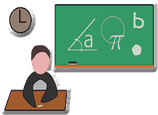Conditional Remix & Share Permitted
CC BY-NC-SA
Rating
0.0 stars

The resource leads to an interactive website where students can learn and practice arithmetic essentials. Currently these are Mean and Median, Exponents, Square Roots and Arithmetic Sequences. I hope to be expanding the website as time allows.

Subject:
Mathematics
Numbers and Operations
Material Type:
Interactive
Lesson
Module
Author:
Jerry Pappas
04/22/2021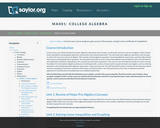Unrestricted Use
CC BY
Rating
0.0 stars

This course is also intended to provide the student with a strong foundation for intermediate algebra and beyond. Upon successful completion of this course, you will be able to: simplify and solve linear equations and expressions including problems with absolute values and applications; solve linear inequalities; find equations of lines; and solve application problems; add, subtract, multiply, and divide various types of polynomials; factor polynomials, and simplify square roots; evaluate, simplify, multiply, divide, add, and subtract rational expressions, and solve basic applications of rational expressions. This free course may be completed online at any time. It has been developed through a partnership with the Washington State Board for Community and Technical Colleges; the Saylor Foundation has modified some WSBCTC materials. (Mathematics 001)

Subject:
Algebra
Mathematics
Material Type:
Full Course
Provider:
The Saylor Foundation
04/16/2012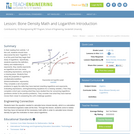Educational Use
Rating
0.0 stars

In their reading from activity 1 of this unit, students should have discovered the term "logarithm." It is at this point that they begin their study of logarithms. Specifically, students examine the definition, history and relationship to exponents; they rewrite exponents as logarithms and vice versa, evaluating expressions, solving for a missing piece. Students then study the properties of logarithms (multiplication/addition, division/subtraction, exponents). They complete a set of practice problems to apply the skills they have learned (rewriting logarithms and exponents, evaluating expressions, solving/examining equations for a missing variable.) Then they complete a short quiz covering what they have studied thus far concerning logarithms (problems similar to the practice problems). They consider how what they have learned moves them closer to answering the unit's challenge question.

Subject:
Applied Science
Engineering
Material Type:
Lesson Plan
Provider:
TeachEngineering
Provider Set:
TeachEngineering
Author:
Kristyn Shaffer
09/18/2014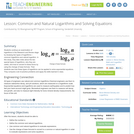Educational Use
Rating
0.0 stars

Students continue an examination of logarithms in the Research and Revise stage by studying two types of logarithms—common logarithms and natural logarithm. In this study, they take notes about the two special types of logarithms, why they are useful, and how to convert to these forms by using the change of base formula. Then students see how these types of logarithms can be applied to solve exponential equations. They compute a set of practice problems and apply the skills learned in class.

Subject:
Applied Science
Engineering
Material Type:
Lesson Plan
Provider:
TeachEngineering
Provider Set:
TeachEngineering
Author:
Kristyn Shaffer
09/18/2014Conditional Remix & Share Permitted
CC BY-NC-SA
Rating
0.0 stars

This is designed to be homework after you teach the interest formula

Subject:
Numbers and Operations
Material Type:
Activity/Lab
Assessment
Homework/Assignment
Author:
Shannay Witte
07/14/2020Unrestricted Use
CC BY
Rating
0.0 stars

The purpose of this task is to introduce the idea of exponential growth and then connect that growth to expressions involving exponents. It illustrates well how fast exponential expressions grow.

Subject:
Mathematics
Material Type:
Activity/Lab
Provider:
Illustrative Mathematics
Provider Set:
Illustrative Mathematics
Author:
Illustrative Mathematics
05/01/2012Some Rights Reserved
Rating
0.0 stars

This math problem demonstrates the concept of geometric progression, through an example of a million dollar contract between an employee and an employer. Application of the concept of geometric progression to social cause activism is addressed. This resource is from PUMAS - Practical Uses of Math and Science - a collection of brief examples created by scientists and engineers showing how math and science topics taught in K-12 classes have real world applications.

Subject:
Geoscience
Mathematics
Physical Science
Material Type:
Lecture Notes
Provider:
NASA
Provider Set:
NASA Wavelength
11/05/2014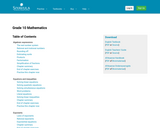Only Sharing Permitted
CC BY-ND
Rating
0.0 stars

This is a comprehensive math textbook for Grade 10. It can be downloaded, read on-line on a mobile phone, computer or iPad. Every chapter has links to on-line video lessons and explanations. Summary presentations at the end of each chapter offer an overview of the content covered, with key points highlighted for easy revision. Topics covered are: algebraic expressions, equations and inequalities, exponents, number patterns, functions, finance and growth, trigonometry, analytical geometry, statistics, probability, Euclidean geometry, measurements. This book is based upon the original Free High School Science Text series.

Subject:
Mathematics
Material Type:
Textbook
Provider:
Siyavula
04/12/2012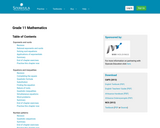Unrestricted Use
CC BY
Rating
0.0 stars

Subject:
Mathematics
Material Type:
Textbook
Provider:
Siyavula
04/12/2012Unrestricted Use
CC BY
Rating
0.0 stars

Subject:
Algebra
Education
Mathematics
Trigonometry
Material Type:
Homework/Assignment
Lecture
Lecture Notes
Lesson
Module
Author:
Linda Neff
08/23/2023Unrestricted Use
CC BY
Rating
0.0 stars

The Factoring module is separated into two parts -- factoring numbers and factoring algebraic expressions.&nbsp; Each part has subtopics. Each subtopic has a video lecture and a practice assignment. &nbsp;The video lecture page includes guided notes in pdf form and videos that follow the guided notes.&nbsp; Each video lecture page also includes supplemental YouTube videos which are optional for students and may be used if further instruction is needed.&nbsp; The practice assignment is a set of exercises in Derivita that correspond to the skills covered in the video lecture.This work, by Magdalene (Maxie) Inigo, is licensed under a&nbsp;Creative Commons Attribution 4.0 International License.Links to an external site.CC-BY

Subject:
Algebra
Education
Mathematics
Trigonometry
Material Type:
Homework/Assignment
Lecture Notes
Lesson
Module
Author:
Linda Neff
08/23/2023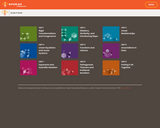Unrestricted Use
CC BY
Rating
0.0 stars

Student-facing 8th grade math resources. Covers transformations & congruence, slope, similarity, linear relationships, associations in data, volume, functions, scientific notation, the pythagorean theorem and irrational numbers.

Subject:
Mathematics
Material Type:
Unit of Study
Provider:
Open Up Resources
05/21/2019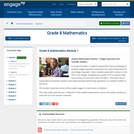Conditional Remix & Share Permitted
CC BY-NC-SA
Rating
0.0 stars

In Grade 8 Module 1, students expand their basic knowledge of positive integer exponents and prove the Laws of Exponents for any integer exponent.  Next, students work with numbers in the form of an integer multiplied by a power of 10 to express how many times as much one is than the other.  This leads into an explanation of scientific notation and continued work performing operations on numbers written in this form.

Find the rest of the EngageNY Mathematics resources at https://archive.org/details/engageny-mathematics.

Subject:
Mathematics
Numbers and Operations
Material Type:
Module
Provider:
New York State Education Department
Provider Set:
EngageNY
05/14/2013Conditional Remix & Share Permitted
CC BY-NC-SA
Rating
0.0 stars

In this classic hands-on activity, learners estimate the length of a molecule by floating a fatty acid (oleic acid) on water. This lab asks learners to record measurements and make calculations related to volume, diameter, area, and height. Learners also convert meters into nanometers. Includes teacher and student worksheets but lacks in depth procedure information. The author suggests educators search the web for more complete lab instructions.

Subject:
Chemistry
Physical Science
Material Type:
Activity/Lab
Provider:
Exploratorium
Author:
Eric Muller
The Exploratorium
11/07/2007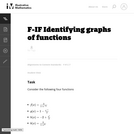Unrestricted Use
CC BY
Rating
0.0 stars

The goal of this task is to get students to focus on the shape of the graph of the equation y=ex and how this changes depending on the sign of the exponent and on whether the exponential is in the numerator or denominator. It is also intended to develop familiarity, in the case of f and k, with the functions which are used in logistic growth models, further examined in ``Logistic Growth Model, Explicit Case'' and ``Logistic Growth Model, Abstract Verson.''

Subject:
Functions
Mathematics
Material Type:
Activity/Lab
Provider:
Illustrative Mathematics
Provider Set:
Illustrative Mathematics
Author:
Illustrative Mathematics
08/17/2012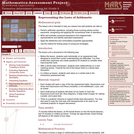Only Sharing Permitted
CC BY-NC-ND
Rating
0.0 stars

This lesson unit is intended to help you assess how well students are able to: Perform arithmetic operations, including those involving whole-number exponents, recognizing and applying the conventional order of operations; Write and evaluate numerical expressions from diagrammatic representations and be able to identify equivalent expressions; apply the distributive and commutative properties appropriately; and use the method for finding areas of compound rectangles.

Subject:
Geometry
Mathematics
Material Type:
Assessment
Lesson Plan
Provider:
Shell Center for Mathematical Education
Provider Set:
Mathematics Assessment Project (MAP)
04/26/2013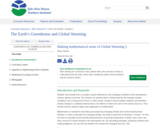Educational Use
Rating
0.0 stars

This unit is designed to give students an introduction to this pressing societal problems and to teach students how to analyze some of the compiled data on global warming through rates, ratios and proportions; students will also learn to make projections and predictions using slope, and linear and exponential functions.

To teach this unit, the teacher has to have at least a general knowledge of global warming, the greenhouse effect, and the carbon cycle. I thought that it was important to explain the basics of these topics. This unit is designed as a math unit, to help students gain a deeper understanding of linear functions, slope, exponential functions, as well as rates, ratios and proportions. Global warming, the carbon cycle, and the greenhouse effect, will be the real life application to which we will apply our mathematics.

Subject:
Applied Science
Environmental Science
Mathematics
Material Type:
Lesson Plan
Unit of Study
Provider:
Yale-New Haven Teachers Institute
Provider Set:
2021 Curriculum Units Volume III